# Three-Way Planar Wilkinsons

Click here to go to our page on the Kouzoujian splitter, a great alternative to the conventional N-way Wilkinson

Three-way planar rat-race coupler (new for October 2017

Three-way branchline (new for October 2017)

This page will provide a basic analysis of planar, three-way Wilkinson splitters in 50 ohm system impedance. This is splitter is imperfect, because it is missing an isolation resistor between the two outer ports, however, this is what makes it easy to lay out. We divided the analysis into three "types" which are described below, and ranked according to bandwidth potential.

### Type 1 splitter

The Type 1 splitter is the simplest network possible. The three arms each employ a single quarter-wave impedance transformer. If you were to impedance match port 1 at center frequency, the transformers would all be 86.6 ohms (transforms each 50 ohm leg to 150 ohms, and three 150 ohms in parallel is 50 ohms). Sorry about the crummy schematic, you'd think by now Agilent would provide a means for graphic capture beyond the usual copy-and-past into Powerpoint, then shrink image with PaintShop...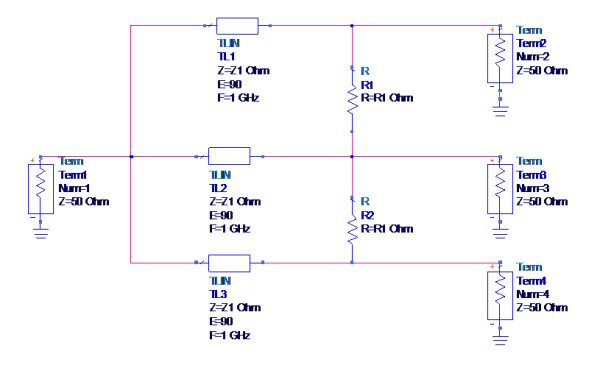The variables R1 and Z1 were allowed to optimize to provide the maximum "-15 dB bandwidth", in other words, it was required that reflection coefficients S11, S22 and S33 and isolation terms S23 and S24 were less than -15 dB. Note that by symmetry, it is not necessary to optimize (or monitor) S44, and S43.

The choice of -15 dB as the goal is arbitrary. However, in the planar three-way Wilkinson, you can't expect perfect isolation of the split ports, and without perfect isolation, you won't get perfect return losses for S22, S33 and S44.

The transmission line impedance values were restricted to no more than 100 ohms (in all three types), to make the circuit realizable in microstrip. It might be better to restrict to a lower value depending on what substrate is chosen, and yield considerations. The subject for another day!

For Type 1, Z1 was found to be 91.4 ohms and R1 was 83.6 ohms, resulting in -15 dB bandwidth of 39%.

Below is the transmission coefficient, showing the near-ideal value of 4.78 dB
[-10*log(1/3)]. All three transmission coefficients are equal in this simplified analysis, in practice the outer branches will behave slightly differently from the center branch.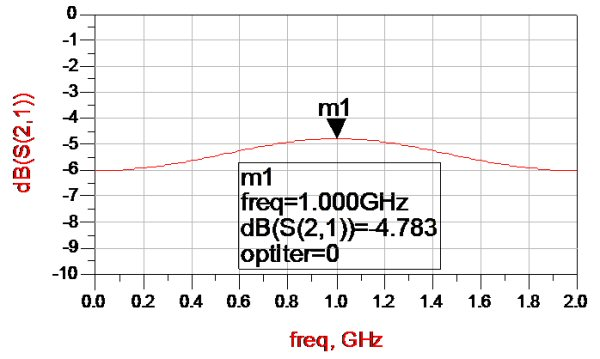Here are the return losses and isolation. Note that all parameters are somewhat compromised, as expected. This is the price that is paid for a planar design with only two isolation resistors.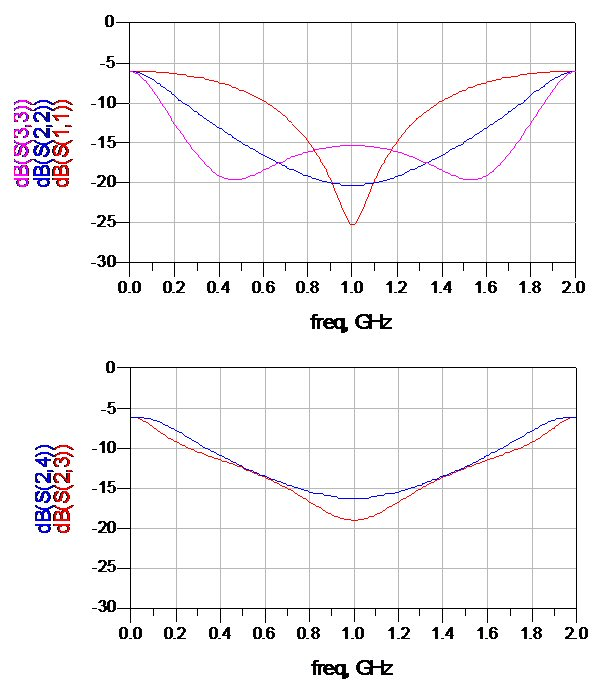### Type 2

In Type 2 we added another transformer section, in this case a single transformer at the input, and component values were reoptimized for maximum -15 dB bandwidth.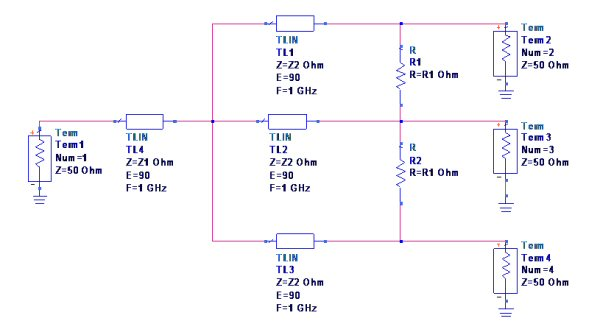The optimized results are shown below. We didn't bother to plot the transmission coefficient, it is similar to that of Type 1. Here, Z1 was found to be 41.9 ohms, Z2 was 91.4 ohms, and R1 was 76.9 ohms, resulting in -15 dB bandwidth of 75%.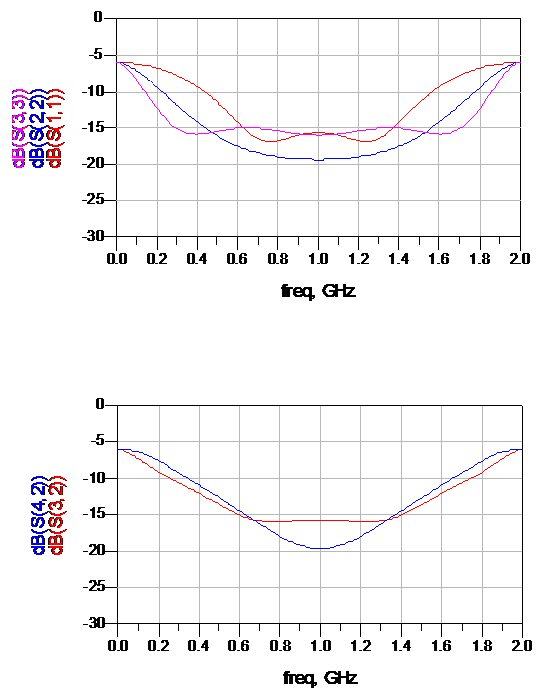### Type 3

In Type 3, the second transformer section was moved to the output ports, and component values were reoptimized for maximum -15 dB bandwidth.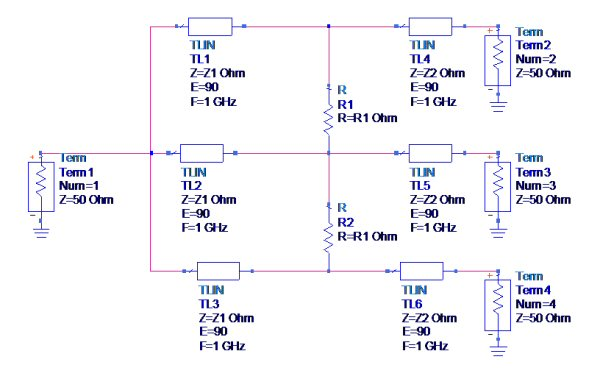The optimized results are shown below. Here, Z1 was found to be 99.3 ohms, Z2 was 54.7 ohms, and R1 was 98.6 ohms, resulting in -15 dB bandwidth of 53%.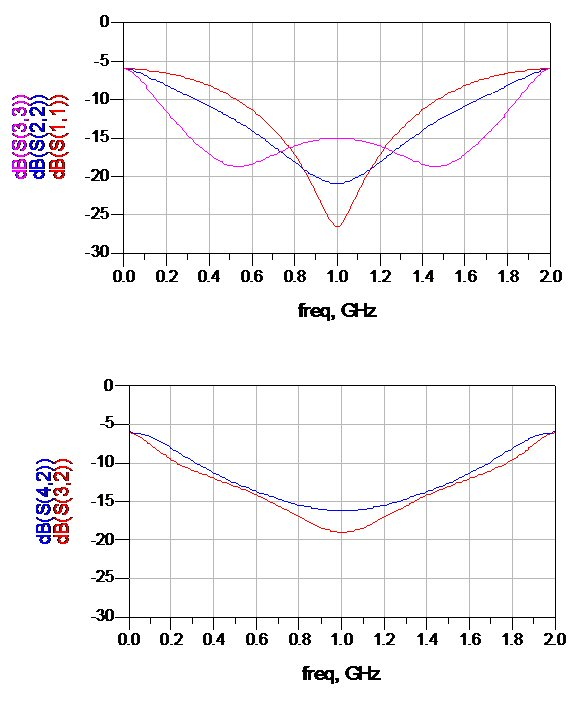In conclusion, Type 2 offers the best bandwidth of the three splitters we examined. Note that if you needed more bandwidth, you could add more sections and a second (or third) set of dump resistors. Planar dividers have been made that span 2-18 GHz in this fashion, and people have been happy to spend \$1000 on them!

### A further example

This example is of a two-stage three-way Wilkinson with two sets of isolation resistors. It was EM-analyzed by some students from India. Bravo!

Below is the linear schematic. We accidentally cropped out the microstrip parameters. The microstrip substrate has ER 4.4, and is 1.5 millimeters in height. We left the conductivity as a PEC (perfect electrical conductor).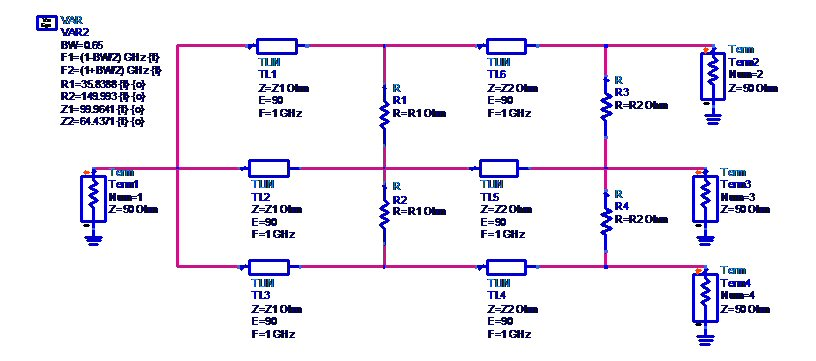And below is the optimization goals and constraints we put on the network. Here we didn't demand 15 dB for the isolation and return losses. We asked for 20 dB, but didn't try to calculate the exact bandwidth that it was capable of, over 65% bandwidth.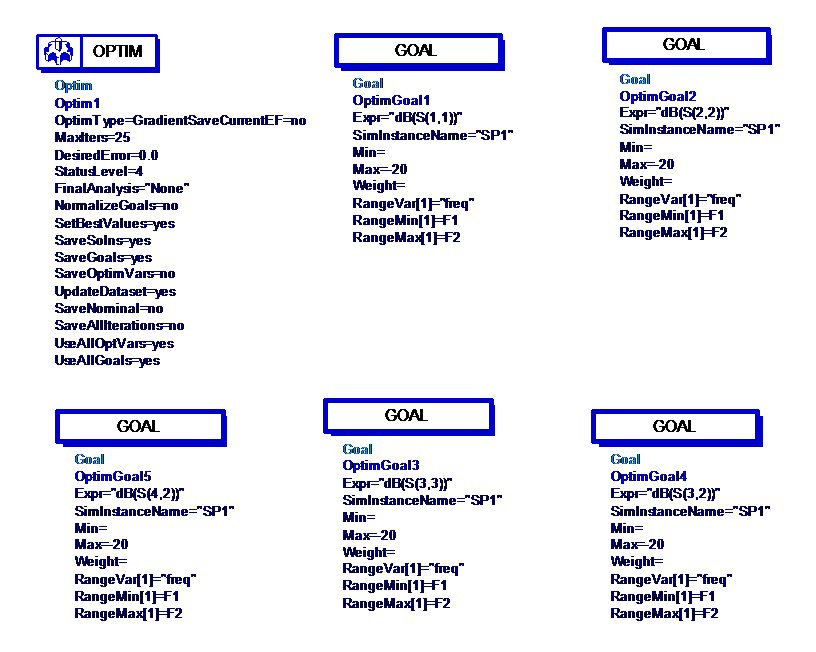Here is the result after optimization (the final values are given in on the schematic). We put a constraint on Z2, it was not allowed to exceed 100 ohms (it is often difficult to realize very high impedances). The parameter that has the least 20-dB bandwidth is S33, which is the return loss of the center leg. Whoops, looks like we also constrained R2 to 150 ohms, there was no reason to put any constraints on the isolation resistors' values.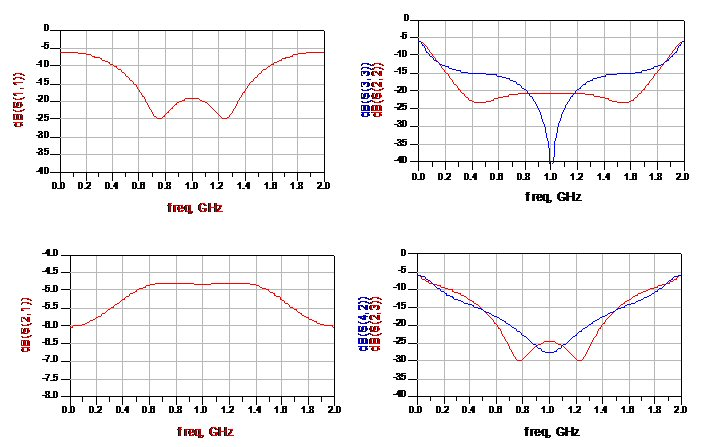What happens when you don't have any resistors in the network? In the schematic below the resistors have all been deactivated.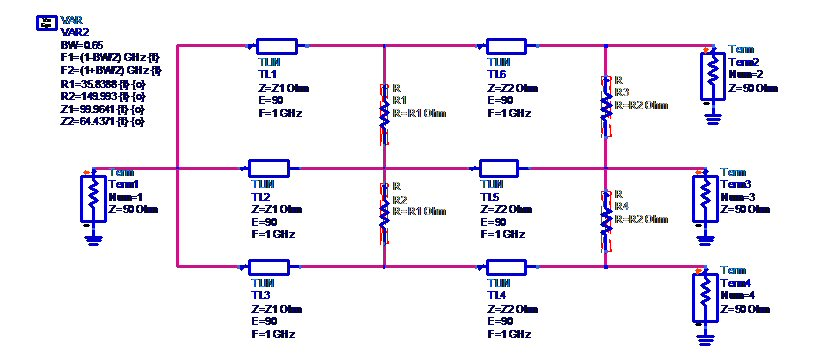The input match (S11) looks the same, while the output matches and isolations have been severely degraded. This is obvious if you study even and odd mode analysis.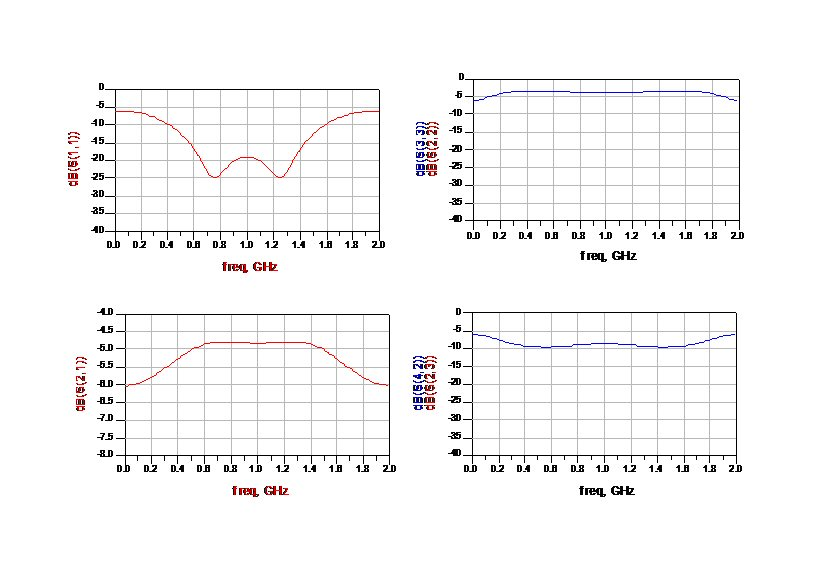Here is the EMed layout of the divider. You can see how the tool divided the network into tiny triangles, upon which Maxwell's Equations are imposed. The resistors (drawn and multi-colored ovals) are not part of the EM analysis, so we don't expect much isolation. The Line lengths in the layout have been folded to achieve approximately 44 mm length to agree with the linear schematic. Why are their elbows in the upper and lower paths? The electrical length through an elbow is some non-zero value. By putting equal number of elbows in each path, you can compensate for them all at once by trimming the three legs' high impedance sections simultaneously. Note that in this layout there are three elbows in the upper and lower paths, and four in the center one, so we slightly violated our intentions...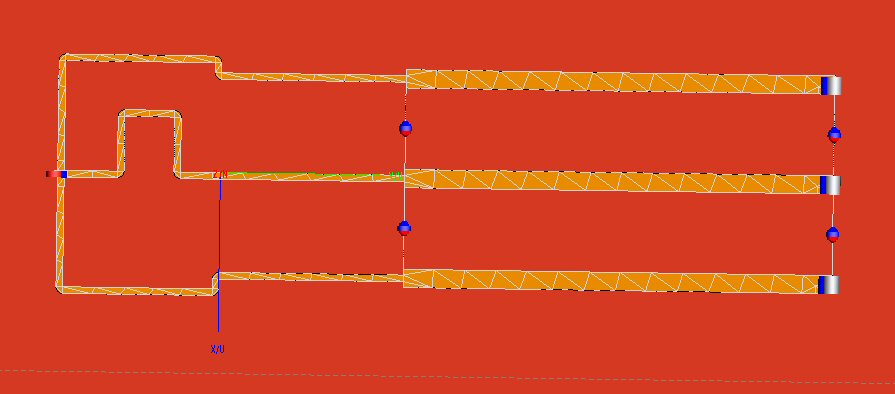And here are some of the parameters, analyzed in an EM tool. Here's the reflection coefficient, which looks similar to the linear analysis but is a more accurate representation of what the circuit will do. Note that it has less return loss at the center of the band than we wanted. Welcome to the real world!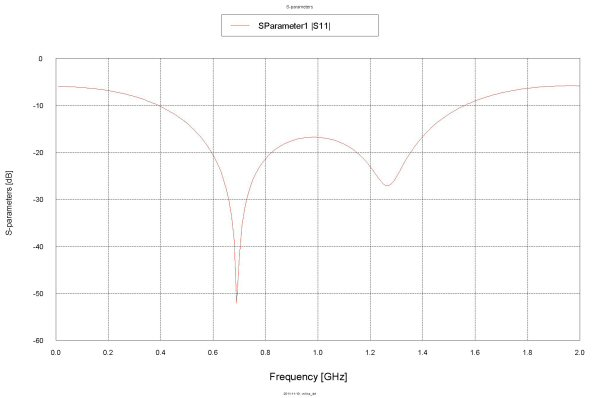And below are the three transmission coefficients, which are remarkable similar. Good job!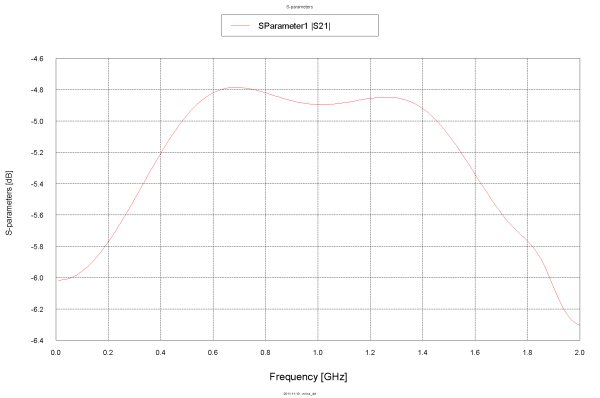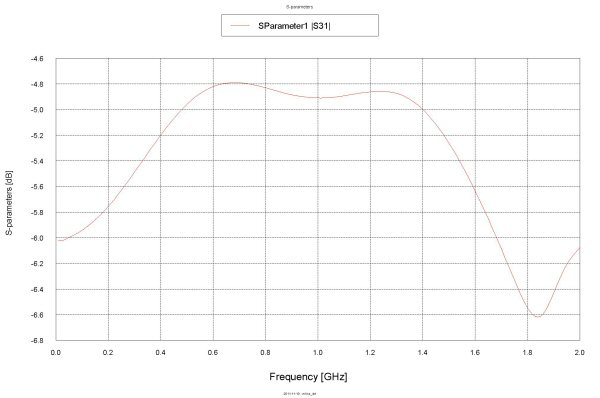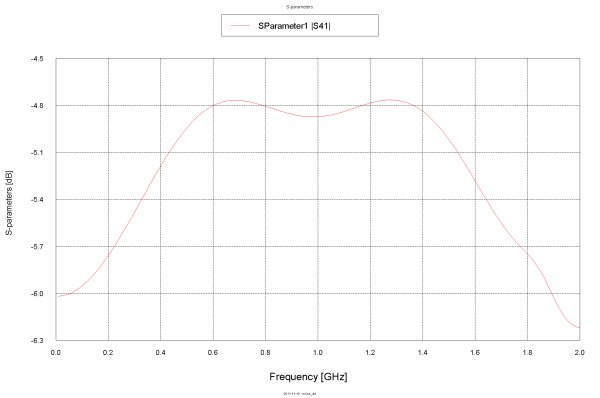### A postulated rule

One of the most basic rules of network theory is for a three-port circuit, you cannot have all ports simultaneously matched if it is lossless (no resistors). We're not going to step out on a limb and say that the same is always true for a four-port (it's not, one example of a matched lossless four-port is a rat-race). But for "related reasons" that we are to lazy to prove, let's postulate that an "N-port Wilkinson-like power divider without resistors" can never be impedance matched at the output ports.

Author : Unknown Editor